×#### Thank you for registering.

One of our academic counsellors will contact you within 1 working day.

Click to Chat

1800-1023-196

+91-120-4616500

CART 0

• 0

MY CART (5)

Use Coupon: CART20 and get 20% off on all online Study Material

ITEM
DETAILS
MRP
DISCOUNT
FINAL PRICE
Total Price: Rs.

There are no items in this cart.
Continue Shopping• Complete JEE Main/Advanced Course and Test Series
• OFFERED PRICE: Rs. 15,900
• View Details

```Chapter 21 Mensuration II (Area of Circle) Exercise – 21.2

Question: 1

Find the area of a circle whose radius is

(i) 7 cm

(ii) 2.1 m

(iii) 7 km

Solution: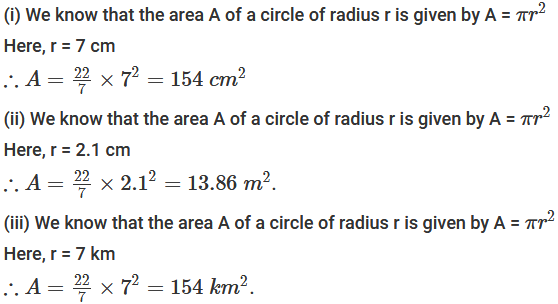Question: 2

Find the area of a circle whose diameter is

(i) 8.4 cm

(ii) 5.6 m

(iii) 7 km

Solution: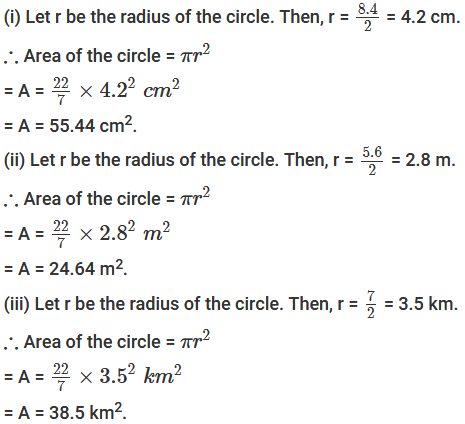Question: 3

The area of a circle is 154 cm2. Find the radius of the circle.

Solution: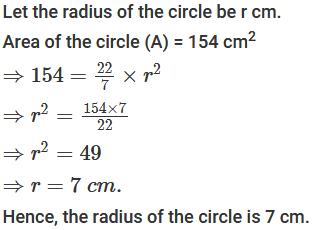Question: 4

Find the radius of a circle, if its area is

(i) 4 it cm2

(ii) 55.44 m2

(iii) 1.54 km2

Solution:Question: 5

The circumference of a circle is 3.14 m, find its area.

Solution: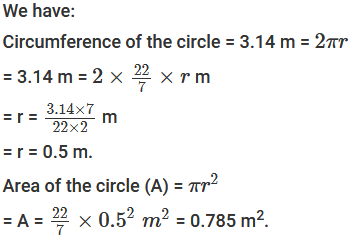Question: 6

If the area of a circle is 50.24 m2, find its circumference.

Solution: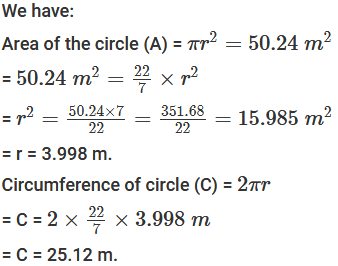Question: 7

A horse is tied to a pole with 28 m long string. Find the area where the horse can graze. (Take π = 22 / 7).

Solution:Question: 8

A steel wire when bent in the form of a square encloses an area of 121 cm2. If the same wire is bent in the form of a circle, find the area of the circle.

Solution: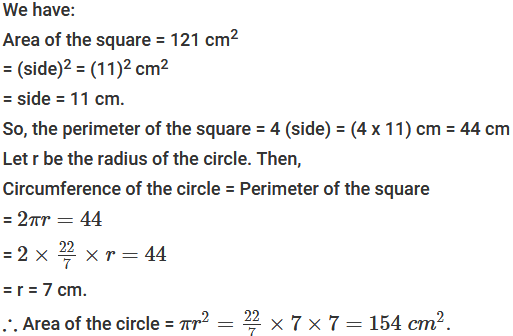Question: 9

A road which is 7 m wide surrounds a circular park whose circumference is 352 m. Find the area of of road.

Solution:Question: 10

Prove that the area of a circular path of uniform width h surrounding a circular region of radius r is πh(2r + h).

Solution: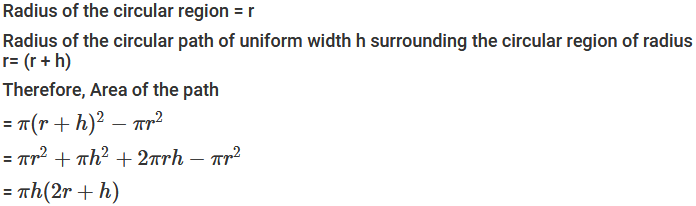Question: 11

The perimeter of a circle is 4πr cm. What is the area of the circle?

Solution: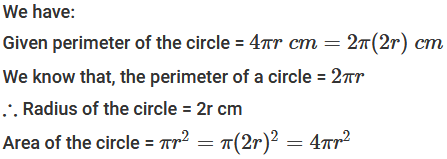Question: 12

A wire of 5024 m length is in the form of a square. It is cut and made a circle. Find the ratio of the area of the square to that of the circle.

Solution: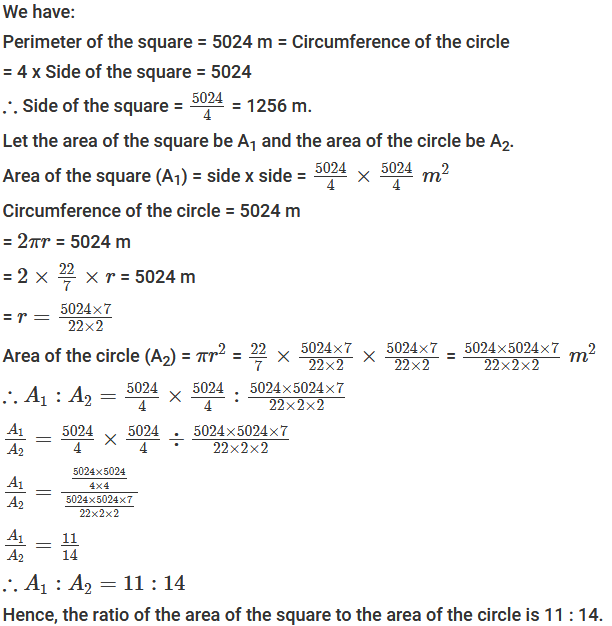Question: 13

The radius of a circle is 14 cm. Find the radius of the circle whose area is double of the area of the circle.

Solution:Question: 14

The radius of one circular field is 20 m and that of another is 48 m. find the radius of the third circular field whose area is equal to the sum of the areas of two fields.

Solution: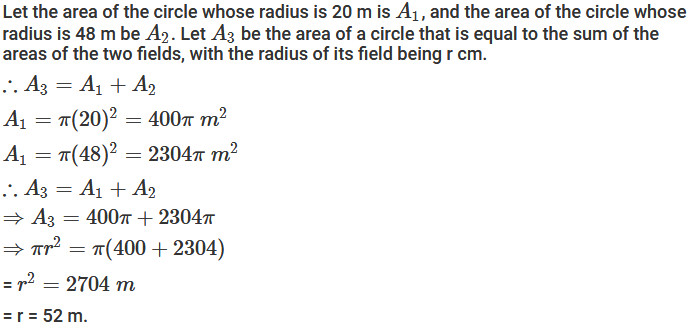Question: 15

The radius of one circular field is 5 m and that of the other is 13 m. Find the radius of the circular field whose area is the difference of the areas of first and second field.

Solution: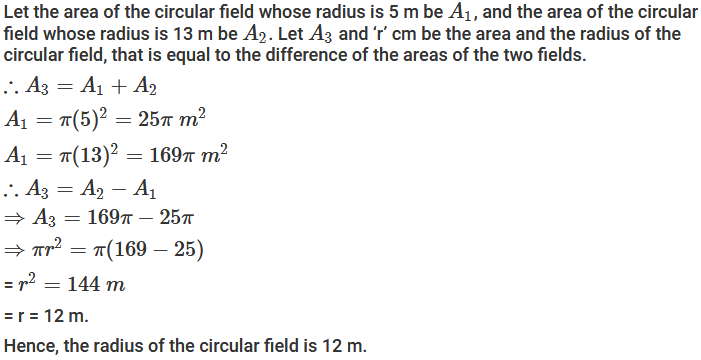Question: 16

Two circles are drawn inside a big circle with diameters 2/3rd and 1/3rd of the diameter of the  big circle as shown in Figure. Find the area of the shaded portion, if the length of the diameter of the circle is 18 cm.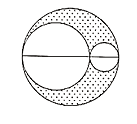Solution: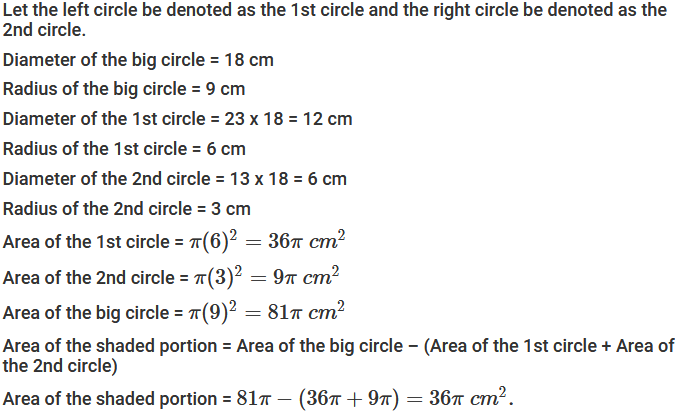Question: 17

In Figure, the radius of quarter circular plot taken is 2 m and radius of the flower bed is 2 m. Find the area of the remaining field.Solution: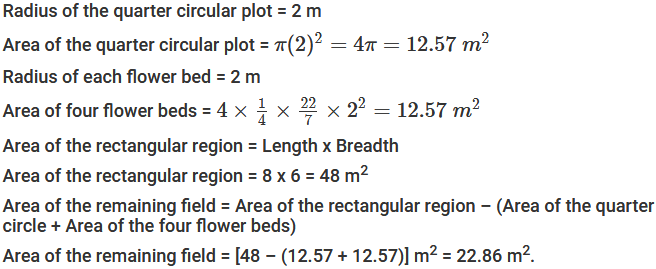Question: 18

Four equal circles, each of radius 5 cm, touch each other as shown in Figure. Find the area included between them. (Take π = 3.14).Solution: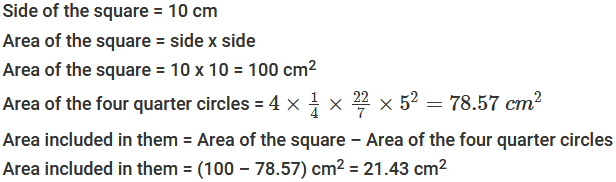Question: 19

The area of circle is 100 times the area of another circle. What is the ratio of their circumferences?

Solution: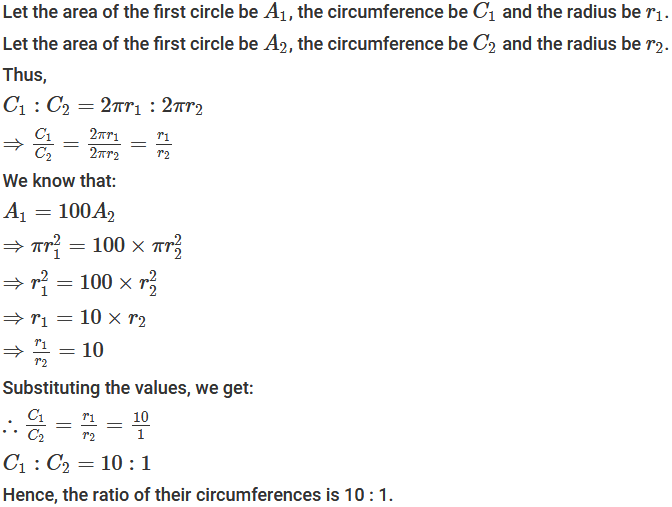```### Course Features

• 728 Video Lectures
• Revision Notes
• Previous Year Papers
• Mind Map
• Study Planner
• NCERT Solutions
• Discussion Forum
• Test paper with Video Solution